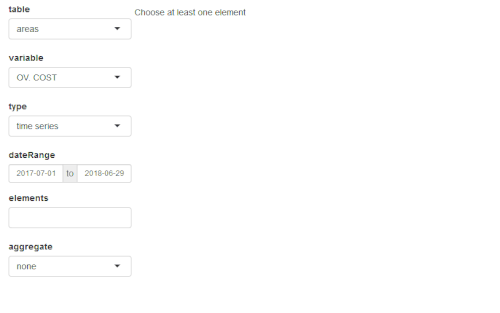Quick presentation of antaresViz

2020-11-13

This package works along with RTE’s adequacy software ANTARES : https://antares-simulator.org/

antaresViz is a package which proposes relevant graphs and maps to vizualize the results of an ANTARES study, with numerous settings and possible customizations.

Where everything starts

library(antaresViz)

The data from an ANTARES study can be imported and easily manipulated using the antaresRead package.

The examples presented below have been build on a fictionnal study whose output have been loaded with antaresRead. They contains different types of elements :

• outputs with an annual and an hourly time step,
• synthetic outputs (averaged on several Monte-Carlo years) and detailled outputs (given year by year),
• outputs for areas, links, clusters and districts.

The aim of this vignette is to give a quick overview of the possibilities offered by the package antaresViz. The data with which the following graphs have been plotted are fictionnal.

The simple power of the plot function

The plot() function, used with an antaresDataList object (i.e. an object returned by the antaresRead::readAntares() function) offers different possible vizualisations.

Moreover, this function is really easy to use. It opens a shiny interface which let the user decide :

• Which type of element he wants to study : areas, links or disctricts.
• Which variable he wants to analyze.
• With which type of graph : barplot, time series, probability density function, heatmap, etc.
• For which area(s), link(s), or district(s).
• And during which time period.
plot(data_hourly_synthesis)Note that all the interface manipulation can also be set directly in the arguments of the plot function. Some examples of the multiple graphs that can be returned by this function are given below.

Barplot of Loss of Load Duration (LOLD) for several districts of the study
plot(
data_annual_synthesis,
table = "districts",
variable = "LOLD",
type = "barplot",
elements = c("00_a", "00_b", "00_c", "00_d",
"00_e", "00_f", "00_g", "00_h", "00_i"),
interactive = FALSE,
width = "100%",
height = 400
)
Time series of the load in an area with average value and 95% confidence interval.
plot(
data_hourly_allmc,
table = "areas",
type = "ts",
elements = "23_b",
confInt = 0.95,
dateRange = c("2018-01-08", "2018-01-14"),
width = "100%",
height = 400,
interactive = FALSE
)
Probability density function of the wind power generation in two areas
plot(
data_hourly_synthesis,
table = "areas",
variable = "WIND",
type = "density",
elements = c("01_a", "02_a"),
interactive = FALSE,
width = "100%",
height = 400
)
Heatmap of the congestion probability of one interconnection
plot(
data_hourly_synthesis_1year,
variable = "CONG. PROB +",
type = "heatmap",
elements = "25_c - 26_d",
interactive = FALSE,
width = "100%",
height = 400,
main = "Congestion probability"
)

Note that the plot() function also contains a compare argument which allows comparisons between :

• different variables
• different studies

When the production meets the demand

The prodStack function builds a graph which contains the time series of demand in one area (or the sum of the demand of a set of areas) along with the generation of this area (or set of areas), divided between the different fuel types (e.g. nuclear, gas, wind, etc).

prodStack(data_hourly_synthesis)

Once again, this function is easy to use and opens a shiny interface which let the user manipulate the selected areas and time range. Some settings can also be passed to the function through its (optionnal) arguments.

prodStack(
data_hourly_synthesis,
stack = "eco2mix",
areas = "37_h",
dateRange = c("2018-01-08", "2018-01-21"),
main = "Production stack",
unit = "GWh",
interactive = FALSE,
width = "100%",
height = 500
)

The graphical template used by default is the one of the RTE’s application eco2mix. This template can though be redefined completely by the user with the function setProdStackAlias().

The exchangesStack() function proposes similar graphs with a superposition of all the imports and exports of an area.

exchangesStack(
data_hourly_synthesis,
area = "37_h",
dateRange = c("2018-01-08", "2018-01-21"),
main = "Import/Export of area 37_h",
unit = "GWh",
interactive = FALSE,
width = "100%",
height = 500
)

Everything looks better on a map

Last but not least, antaresViz proposes several function to vizualise the results of a study on a map.

To do so, the first function to use is mapLayout(). This function launches an interactive application that let the user place areas of the ANTARES study on a map.